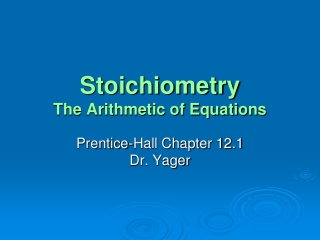DownloadDownload PresentationStoichiometry The Arithmetic of Equations

# Stoichiometry The Arithmetic of Equations

Download Presentation## Stoichiometry The Arithmetic of Equations

- - - - - - - - - - - - - - - - - - - - - - - - - - - E N D - - - - - - - - - - - - - - - - - - - - - - - - - - -
##### Presentation Transcript

1. StoichiometryThe Arithmetic of Equations Prentice-Hall Chapter 12.1 Dr. Yager

2. Objectives • Explain how balanced equations apply to both chemistry and everyday situations. • Interpret balanced chemical equations in terms of moles, representative particles, mass, and gas volume at STP. • Identify the quantities that are always conserved in chemical reactions.

3. A balanced chemical equation provides the same kind of quantitative information that a recipe does.

4. Here is the Problem! • Cupcake Recipe for 10 cupcakes: • 2 eggs • 3 cups of flour • 1 cup sugar • You have: • 3 eggs • 4 cups of flour • 2 cups of sugar • What limits the number of cupcakes you can make?

5. The original recipe is: 2:3:1 2 eggs, 3 C flour, 1 C sugar Given: 3 eggs, 4 C flour, 2 C sugar • 3 eggs requires: 4.5 cups of flour and 1.5 cups of sugar • 4 cups of flour requires: 2 2/3 eggs and 1 1/3 cups of sugar • 2 cups of sugar requires: 4 eggs and 6 cups of flour The only one possible is #2!

6. The Chemist: Uses the ratio or balanced equation to calculate how muchreactant is needed or product formed. The calculation of quantities in chemical reactions is a subject of chemistry called stoichiometry.

7. Stoichiometry • Defined as the calculation of quantities in chemical reactions • Form of bookkeeping for chemists • Generally, one of the reactants limits the calculations

8. A balanced chemical equation can be interpreted in terms of different quantities, including numbers of atoms, molecules, or moles; mass; and volume.

9. N2(g) + 3H2(g) 2NH3(g) Interpreting Chemical Equations

10. Atoms, Molecules, etc..

11. Moles

12. Mass

13. Volume

14. What is Conserved? • Mass and atoms are conserved. • Molecules, formula units, moles and volumes are not necessarily conserved. • Example where they are conserved: H2(g) + I2(g) 2HI(g)

15. Balance and Tell What is Conserved C2H2 (g) + O2 (g) CO2 (g) + H2O(g) 2C2H2(g) + 5O2(g) 4CO2(g) + 2H2O(g) Atoms – Yes Mass - Yes Molecules – No Formula Units – No Moles – No Volume – No

16. 1. A manufacturer of bicycles has 5350 wheels, 3023 frames, and 2655 handlebars. How many bicycles can be manufactured using these parts? • 2675 bicycles • 2655 bicycles • 3023 bicycles • 5350 bicycles

17. 1. A manufacturer of bicycles has 5350 wheels, 3023 frames, and 2655 handlebars. How many bicycles can be manufactured using these parts? • 2675 bicycles • 2655 bicycles • 3023 bicycles • 5350 bicycles

18. 2. A reaction that produces iron metal from iron ore: Fe2O3•H2O(s) + 3CO(g)  2Fe(s) + 3CO2(g) + H2O(g) What is the volume of gas at STP that reacts and the volume of gas at STP produced? • 3 L and 4 L. • 67.2 L and 89.6 L. • 67.2 L and 67.2 L • 3 L and 3 L

19. 2. A reaction that produces iron metal from iron ore: Fe2O3•H2O(s) + 3CO(g)  2Fe(s) + 3CO2(g) + H2O(g) What is the volume of gas at STP that reacts and the volume of gas at STP produced? • 3 L and 4 L. • 67.2 L and 89.6 L. • 67.2 L and 67.2 L • 3 L and 3 L

20. 3. What is conserved in this reaction? H2(g) + Cl2(g)  2HCl(g) • only mass • only mass and number of moles • only mass, number of moles, and number of molecules • mass, number of moles, number of molecules, and volume

21. 3. What is conserved in this reaction? H2(g) + Cl2(g)  2HCl(g) • only mass • only mass and number of moles • only mass, number of moles, and number of molecules • mass, number of moles, number of molecules, and volume